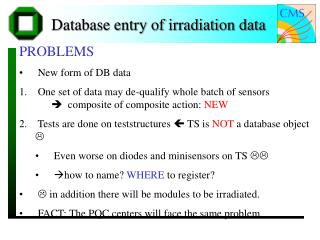DownloadDownload Presentation# Database entry of irradiation data

Télécharger la présentation## Database entry of irradiation data

- - - - - - - - - - - - - - - - - - - - - - - - - - - E N D - - - - - - - - - - - - - - - - - - - - - - - - - - -
##### Presentation Transcript

1. Database entry of irradiation data • PROBLEMS • New form of DB data • One set of data may de-qualify whole batch of sensors  composite of composite action: NEW • Tests are done on teststructures  TS is NOT a database object  • Even worse on diodes and minisensors on TS  • how to name? WHERE to register? •  in addition there will be modules to be irradiated. • FACT: The PQC centers will face the same problem

2. Steps to go, and possible solution • We need a discussion with Didier • The composite of composite action has to be introduced in the DB scheme • IQC and also PQC tables could be inserted in the QTC existing DB objects • Addition of new tables • Optional, since only a fraction will be irradiated • M200 and pre-series is approaching fast!

3. Irrad tables already exist in XML • Tables are done and already underwent one iteration between Karlsruhe and Louvain • We will have 3 simple tables: • diodeminisensorsensor • Tables show the results from the agreed measurements • Ramps, mean values, difference of values before and after irradiation

4. DB content (for sensor & minissensor) • ENV: Date,Time;Irrad_Date;Comment;Humidity,Temp Duration; operator; location; status • GLOBAL: I_TOT, V_dep; C_TOT; alpha (before and after irrad) • IRRAD-SPECS: fluence(p,n); dose(p,n); energy(p,n) • STRIP-PARAMETERS: mean[Rpoly;Cint;Ileak;Rint (CAC optional)]@Vstandard Delta of all strip parameters before and after irrad • Raw Data: IV,CV, ONE RAMP {Rpoly;Cint;Ileak;Rint (CAC optional)} vs. Voltage

5. Tables for diode Tables for sensors and minisensors are similar but more extensive.

6. Addition for sensor and minisensor <result name="Rpoly_mean_V1_MOhm" value="175.44"/> <result name="Rpoly_delta_V1_MOhm" value=".10"/> <result name="I_strip_nA" value="552.00"/> <result name="I_strip_delta_nA" value="0"/> <result name="C_ac_mean_V1_pF" value="552.00"/> <result name="C_ac_delta_V1_pF" value="0"/> <result name="C_int_1Mhz_mean_V1_pF" value="552.00"/> <result name="C_int_1MHz_delta_V1_pF" value="552.00"/> <result name="R_int_mean_V1_MOhm" value="552.00"/> <result name="R_int_delta_V1_MOhm" value="552.00"/> <result name="C_tot_pF" value="22530.00 21370.00 ..... 1855.00 1855.00"/> <result name="Rpoly_MOhm" value="52.10 95.48 ...... 194.36 194.94"/> <result name="C_int_1MHz_pF" value="52.10 95.48 .... 194.36 194.94"/> <result name="I_strip_nA" value="0.32 0.33 ...... 0.32 0.29"/> <result name="C_ac_100Hz_pF" value="52.10 95.48 ........194.36 194.94"/> <result name=„R_int_MOhm" value=„0.68 1.38........100.00100.00"/> </action>

7. Pending Actions • Agreement how to label tables for diode, sensor, minisensor!?! Is the diode, the irrad. Sensor and the minisensor a component of the sensor (same ID)? Are they seperate objects; where will they be registered? • @DB people implemtation of the composite of composites • Implementation of the agreed! Tables into the DB (discussion underway) • Labview vi for the XML output (I volonteer)